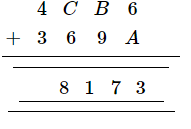# Replace A, B, C by suitable numerals.

Question:

Replace A, B, C by suitable numerals.Solution:

$A=7, A+6=7+6=13 \quad$ (1 is carried over)

$(1+B+9)=17$, or $B=7 \quad$ ( 1 is carried over)

$A=7, B=7$ and $C=4 \quad(1$ is carried over $)$

$\therefore A=7, B=7$ and $C=4$